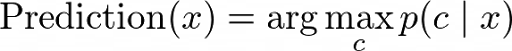Naive Bayes classification - Tech It Yourself

## Friday, 23 April 2021

1. Usage

• NBC is extremely fast for both training and prediction

• NBC is often very easily interpretable

• NBC has very few (if any) tunable parameters

When the data match naive assumptions (very rare in practice)

• For very well-separated categories, and simple model is needed

• For very high-dimensional data, and simple model is needed

2. Implementation

Classes c₁, c₂, c

Features x₁, x

The result of a classifier isp(c) is the probability (frequencies) that class c is observed in the labeled dataset.
With assumption x₁, x₂ are independent
how to model p(x₁|c₁), p(x₂|c₁), p(x₁|c₂), p(x₂|c₂), p(x₁|c₃) and p(x₂|c₃)?
If the features are 0 and 1 only, you could use a Bernoulli distribution.
If the features are integers, a Multinomial distribution.
If the features are real values, a Gaussian distribution.

With a class cⱼ from the data, estimates μᵢ,ⱼ (the mean) and σᵢ,ⱼ  (the standard deviation) for each feature i.

import numpy as np
import matplotlib.pyplot as plt
import seaborn as sns
sns.set()
from sklearn.datasets import make_blobs

X, y = make_blobs(n_samples=20, centers=[(0,0), (4,4), (-4, 4)], random_state=2)
print(X.shape)
plt.scatter(X[:, 0], X[:, 1], c=y, s=50, cmap='RdBu');
plt.show()

class GNB:
def __init__(self):
pass
def fit(self, X, y):
print(y)
total = len(y)
self.unique_y = np.unique(y)
self.params = {}
for j in self.unique_y:
id_class_j = np.where(y==j)
prob_class_j = len(id_class_j)/total
x_class_j = X[id_class_j]
mean_class_j = np.mean(x_class_j, axis=0)
std_class_j = np.std(x_class_j, axis=0)
self.params[j] = [prob_class_j, mean_class_j, std_class_j]

def find_prob(self, X):
probs = []
for x in X:
prob = []
for j in self.unique_y:
prob_class_j, mean_class_j, std_class_j = self.params[j]
pij = (1/np.sqrt(2 * np.pi * std_class_j **2)) * np.exp((-1/2) * ((np.array(x) - mean_class_j)/std_class_j) **2)
pij = np.prod(pij)
pij *= prob_class_j
prob.append(pij)
prob = np.array(prob)
pij_sum = np.sum(prob)
prob /= pij_sum
probs.append(prob)

return probs

my_gauss = GNB()
my_gauss.fit(X, y)
rrs = my_gauss.find_prob([[-2, 5], [0,0], [6, -0.3]])

for r in rrs:
print(r)